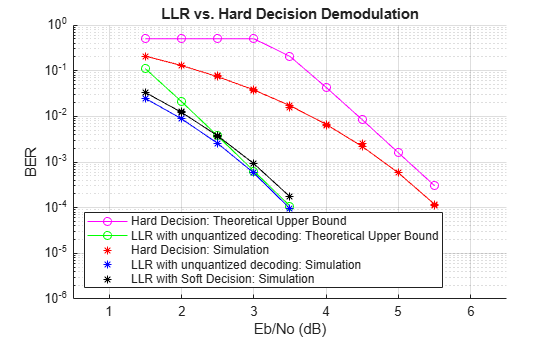# Log-Likelihood Ratio (LLR) Demodulation

This example shows the BER performance improvement for QPSK modulation when using log-likelihood ratio (LLR) instead of hard-decision demodulation in a convolutionally coded communication link. With LLR demodulation, one can use the Viterbi decoder either in the unquantized decoding mode or the soft-decision decoding mode. Unquantized decoding, where the decoder inputs are real values, though better in terms of BER, is not practically viable. In the more practical soft-decision decoding, you quantize the demodulator output before sending it to the decoder. It is generally observed that soft-decision decoding does not incur a significant cost in BER while significantly reducing the decoder complexity. This example validates the BER performance through simulation.

For a Simulink™ version of this example, see LLR vs. Hard Decision Demodulation in Simulink.

### Initialization

Initialize simulation parameters.

```M = 4; % Modulation order bitsPerIter = 1.2e4; % Number of bits to simulate EbNo = 3; % Information bit Eb/No in dB```

Initialize coding properties for a rate 1/2, constraint length 7 code.

```codeRate = 1/2; % Code rate of convolutional encoder constLen = 7; % Constraint length of encoder codeGenPoly = [171 133]; % Code generator polynomial of encoder tblen = 32; % Traceback depth of Viterbi decoder trellis = poly2trellis(constLen,codeGenPoly);```

Create a `comm.ConvolutionalEncoder` System object™ by using `trellis` as an input.

`enc = comm.ConvolutionalEncoder(trellis);`

Channel

The signal going into the AWGN channel is the modulated encoded signal. To achieve the required noise level, adjust the Eb/No for coded bits and multi-bit symbols. Calculate the $SNR$ value based on the ${E}_{b}/{N}_{o}$ value you want to simulate.

`SNR = convertSNR(EbNo,"ebno","BitsPerSymbol",log2(M),"CodingRate",codeRate);`

Viterbi Decoding

Create `comm.ViterbiDecoder` objects to act as the hard-decision, unquantized, and soft-decision decoders. For all three decoders, set the traceback depth to `tblen`.

```decHard = comm.ViterbiDecoder(trellis,'InputFormat','Hard', ... 'TracebackDepth',tblen); decUnquant = comm.ViterbiDecoder(trellis,'InputFormat','Unquantized', ... 'TracebackDepth',tblen); decSoft = comm.ViterbiDecoder(trellis,'InputFormat','Soft', ... 'SoftInputWordLength',3,'TracebackDepth',tblen);```

Calculating the Error Rate

Create `comm.ErrorRate` objects to compare the decoded bits to the original transmitted bits. The Viterbi decoder creates a delay in the decoded bit stream output equal to the traceback length. To account for this delay, set the `ReceiveDelay` property of the `comm.ErrorRate` objects to `tblen`.

```errHard = comm.ErrorRate('ReceiveDelay',tblen); errUnquant = comm.ErrorRate('ReceiveDelay',tblen); errSoft = comm.ErrorRate('ReceiveDelay',tblen);```

### System Simulation

Generate `bitsPerIter` message bits. Then convolutionally encode and modulate the data.

```txData = randi([0 1],bitsPerIter,1); encData = enc(txData); modData = pskmod(encData,M,pi/4,InputType="bit");```

Pass the modulated signal through an AWGN channel.

`[rxSig,nVar] = awgn(modData,SNR);`

Before using a `comm.ViterbiDecoder` object in the soft-decision mode, the output of the demodulator needs to be quantized. This example uses a `comm.ViterbiDecoder` object with a `SoftInputWordLength` of 3. This value is a good compromise between short word lengths and a small BER penalty. Define partition points for 3-bit quantization.

```demodLLR.Variance = nVar; partitionPoints = (-1.5:0.5:1.5)/nVar;```

Demodulate the received signal and output hard-decision bits.

`hardData = pskdemod(rxSig,M,pi/4,OutputType="bit");`

Demodulate the received signal and output LLR values.

`LLRData = pskdemod(rxSig,M,pi/4,OutputType="llr",NoiseVariance=nVar);`

Hard-decision decoding

Pass the demodulated data through the Viterbi decoder. Compute the error statistics.

```rxDataHard = decHard(hardData); berHard = errHard(txData,rxDataHard);```

Unquantized decoding

Pass the demodulated data through the Viterbi decoder. Compute the error statistics.

```rxDataUnquant = decUnquant(LLRData); berUnquant = errUnquant(txData,rxDataUnquant);```

Soft-decision decoding

Pass the demodulated data to the `quantiz` function. This data must be multiplied by `-1` before being passed to the quantizer, because, in soft-decision mode, the Viterbi decoder assumes that positive numbers correspond to 1s and negative numbers to 0s. Pass the quantizer output to the Viterbi decoder. Compute the error statistics.

```quantizedValue = quantiz(-LLRData,partitionPoints); rxDataSoft = decSoft(double(quantizedValue)); berSoft = errSoft(txData,rxDataSoft);```

### Running Simulation Example

Simulate the previously described communications system over a range of Eb/No values by executing the simulation file `simLLRvsHD`. It plots BER results as they are generated. BER results for hard-decision demodulation and LLR demodulation with unquantized and soft-decision decoding are plotted in red, blue, and black, respectively. A comparison of simulation results with theoretical results is also shown. Observe that the BER is only slightly degraded by using soft-decision decoding instead of unquantized decoding. The gap between the BER curves for soft-decision decoding and the theoretical bound can be narrowed by increasing the number of quantizer levels.

This example may take some time to compute BER results. If you have the Parallel Computing Toolbox™ (PCT) installed, you can set `usePCT` to `true` to run the simulation in parallel. In this case, the file `LLRvsHDwithPCT` is run.

To obtain results over a larger range of Eb/No values, modify the appropriate supporting files. Note that you can obtain more statistically reliable results by collecting more errors.

```usePCT = false; if usePCT && license('checkout','Distrib_Computing_Toolbox') ... && ~isempty(ver('parallel')) LLRvsHDwithPCT(1.5:0.5:5.5,5); else simLLRvsHD(1.5:0.5:5.5,5); end```### Appendix

The following functions are used in this example: